# Python Arrays

Challenge Inside! : Find out where you stand! Try quiz, solve problems & win rewards!
Learn via video coursePython Course for Beginners With Certification: Mastering the Essentials
By Rahul JanghuFree
4.90Enrolled: 1000Python Course for Beginners With Certification: Mastering the Essentials
Rahul JanghuFree
4.90
Enrolled: 1000

## Overview

An array in python is defined as a container that stores the collection of items at contiguous memory locations. The items that are stored in the array should be of the same type. Python arrays are dynamic. As we know that arrays in python are mutable which means we can change the original array at any point in time.

## Introduction

Ever wondered how you can store marks of different subjects of a student and then update it (if required). So answer to this problem lies inside arrays of python. Arrays can store as many of data into a single variable. Read along to know more.

## What is Array?

An array is defined as a container that stores the collection of items at contiguous memory locations. The items that are stored in array should be of the same type. The array is an idea of storing multiple items of the same type together and it makes it easier to calculate the position of each element. It is used to store multiple values in a single variable. Python arrays are much faster than list as it uses less memory.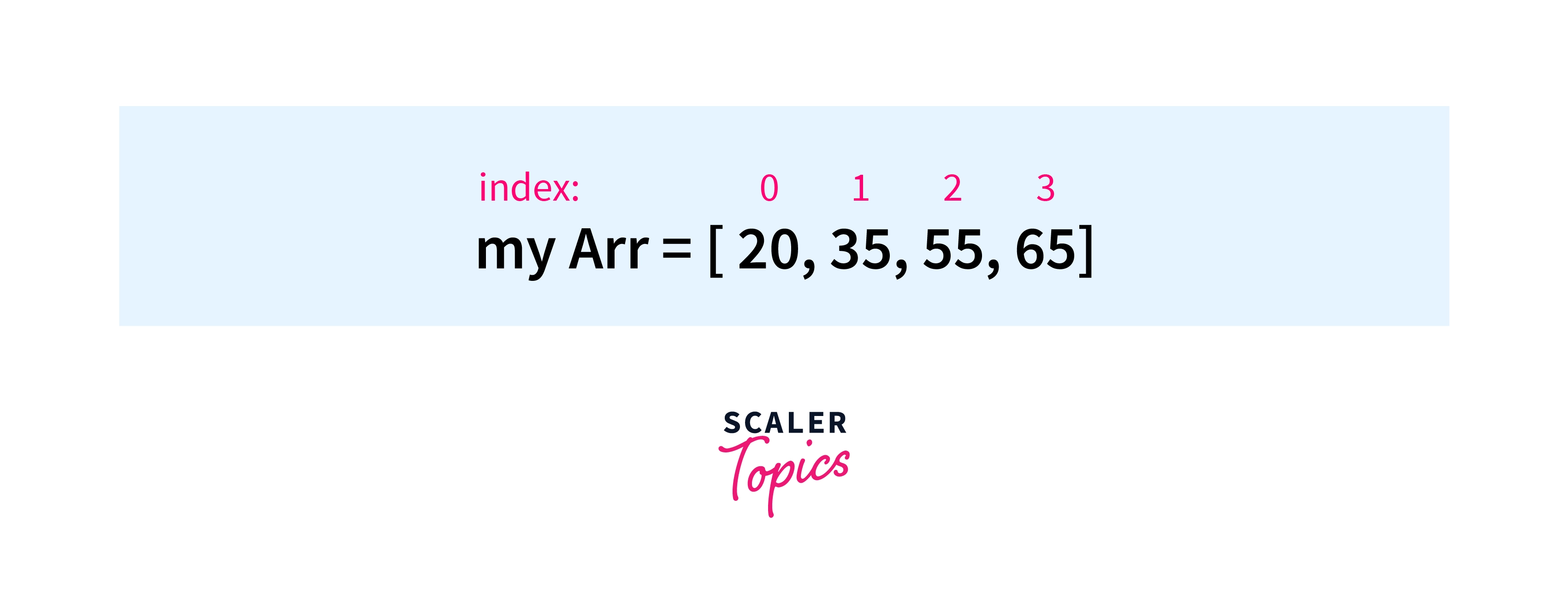As we know, Python arrays are dynamic in nature so we don't need to specify the size of the array at the beginning of the code. We can have as many of the elements inside the array.

As we have studied earlier, arrays are mutable so we can update the array at any point in time. We can add an element to the existing array, delete any of the elements, even update the particular index with the new values.

There are several features and methods which are used on arrays for searching, deleting, adding an element, etc. Read along to know more.

## Creating an Array in Python

For creating an array in Python, we need to import the array module.

After importing, the array module we just have to use the array function which is used to create the arrays in Python.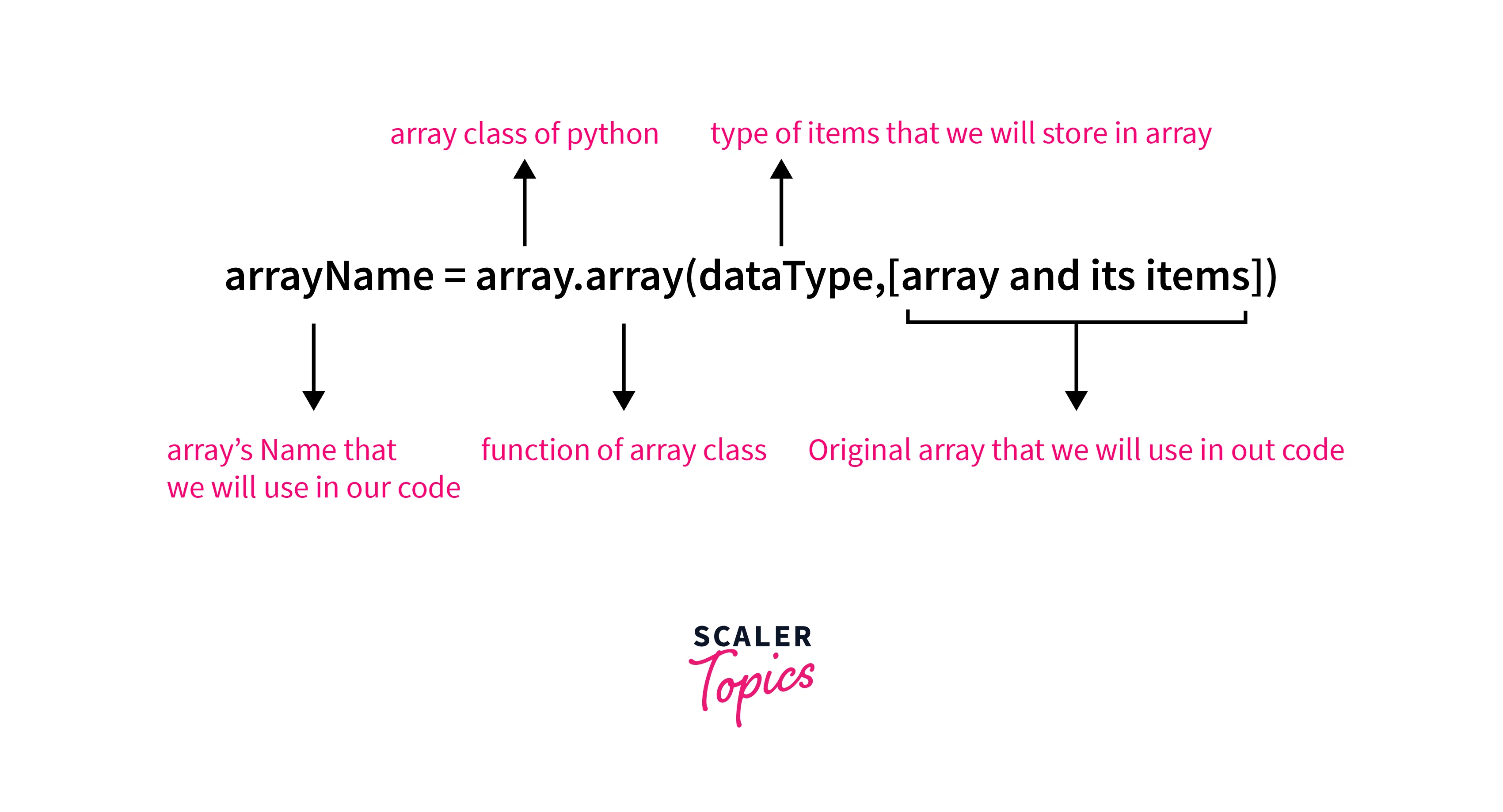Syntax

Code for Data Types which are used in array Function

Code TypePython TypeFull FormSize(in Bytes)
uunicode characterPython Unicode2
bintSigned Char1
BintUnsigned Char1
hintSigned Short2
lintSigned Long4
LintUnsigned Long4
qintSigned Long Long8
QintUnsigned Long Long8
HintUnsigned Short2
ffloatFloat4
dfloatDouble8
iintSigned Int2
IintUnsigned Int2

Example

Here, we have created an array with the name myArr and printed it to get the output.

Output

Explanation

First of all, we have imported array class in our code. After that, we passed 2 parameters to the array function where the first one is the data type in which we want to create an array and the other is the items of the array which we need inside it. Finally, we printed the array of `double type which has different elements in it.

## Adding Element to Array in Python

As we all know, arrays are mutable in nature. So we can add an element to the existing array.

We can use 2 different methods to add the elements to the existing array. These methods are:

• .append(): This is used to add a single element to the existing array. By using the append method the element gets added at the end of the existing array.
• .extend(): This is used to add an array to the existing array. By using the extend method the array that we have added to the extend function gets merged at the end of the existing array. And, finally, we got the updated array where we have the combination of the original and the new array.

Let's checkout with the help of an example of how these functions are used to add elements at the end of the array.

Syntax

Example

This code helps us to understand how we can use .append() and .extend(), to add the elements in the array.

Output

The above code adds the elements to the end of the existing array.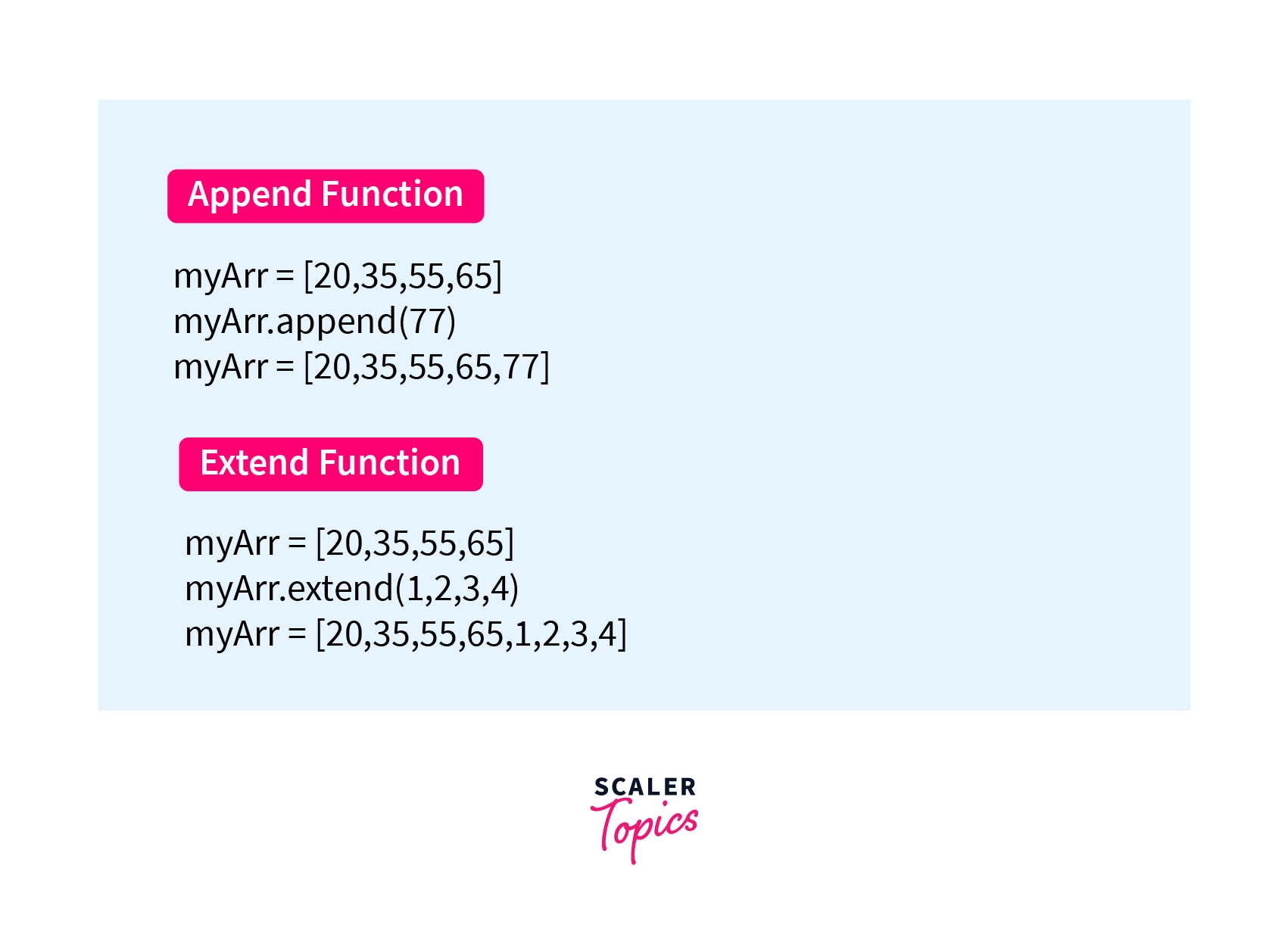Explanation

We have imported the array class into our code. Then we have created the array in which we have stored different values. After that, we have used 2 different functions append() & extend() for adding the elements at the end of the array.

## Accessing Elements from Array in Python

We can access the elements of the array by using the index of the elements of the array.

Note: If we are trying to access that index of the array which is greater than the last index of the array then it will raise the out of bound error.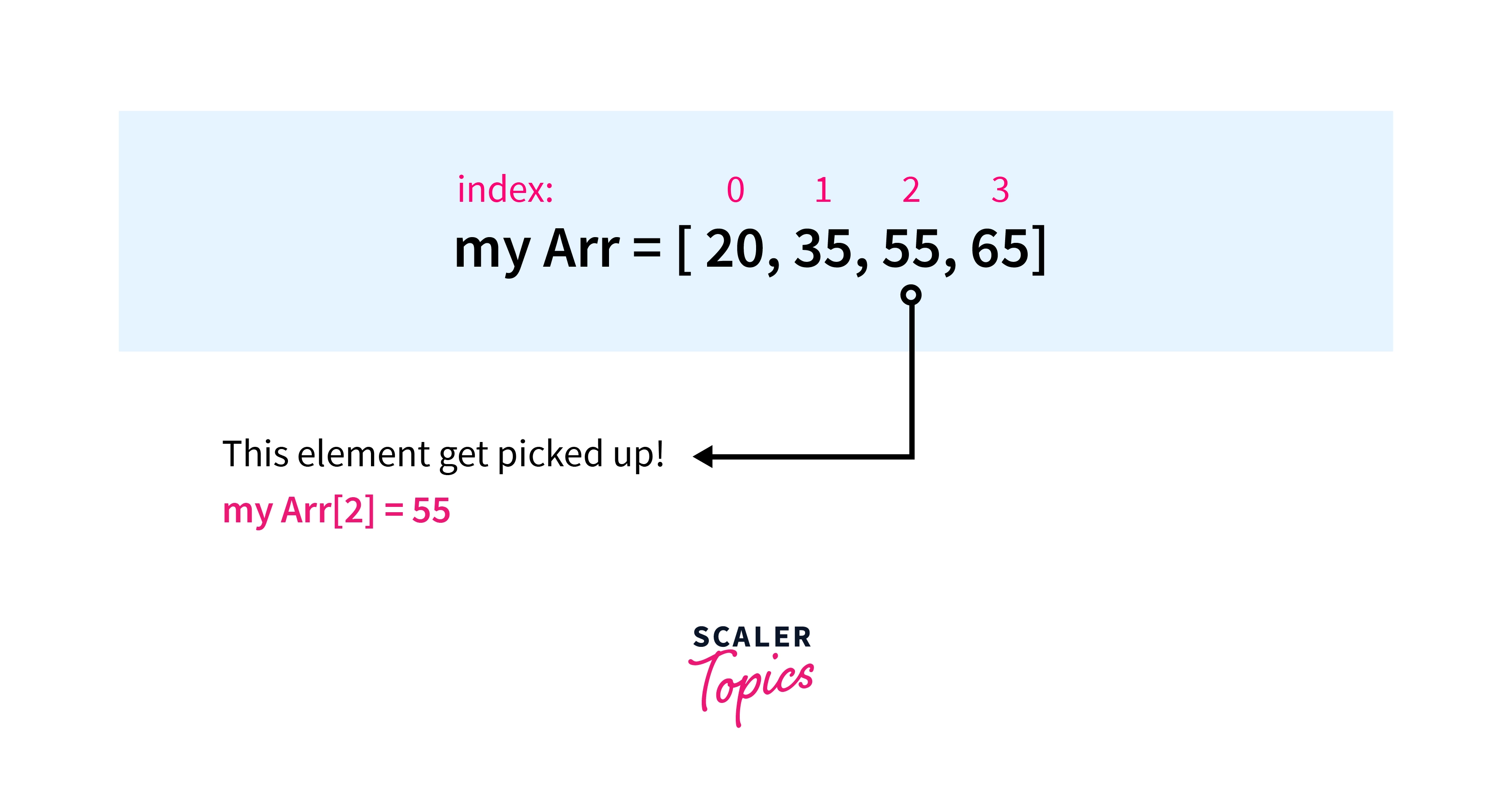Example

This code access the element of the array at a particular index.

Output

The above code prints the element at the 2nd index of the array.

Explanation

First of all, we have imported the array class into our code and then create an array that has different values/elements. Then we access the element using their index positions and stored them into the variable. Finally, we print that variable to get the value at that specific index.

## Removing Elements from Array in Python

We can use the .remove() and pop() functions to remove the elements from the array.

• The pop function deletes the element which is present at the last index of the array.
• The remove function takes an element as a parameter that should be removed from the array. If there are duplicates of the same element which is to be removed from the array, then it will remove the first occurrence of that element.

Note: If we pass an element that is not present in the array as a parameter to the remove() then it will raise a value error i.e that particular element, not in the array. After running this method, the array items are re-arranged, and the index of the elements is re-assigned.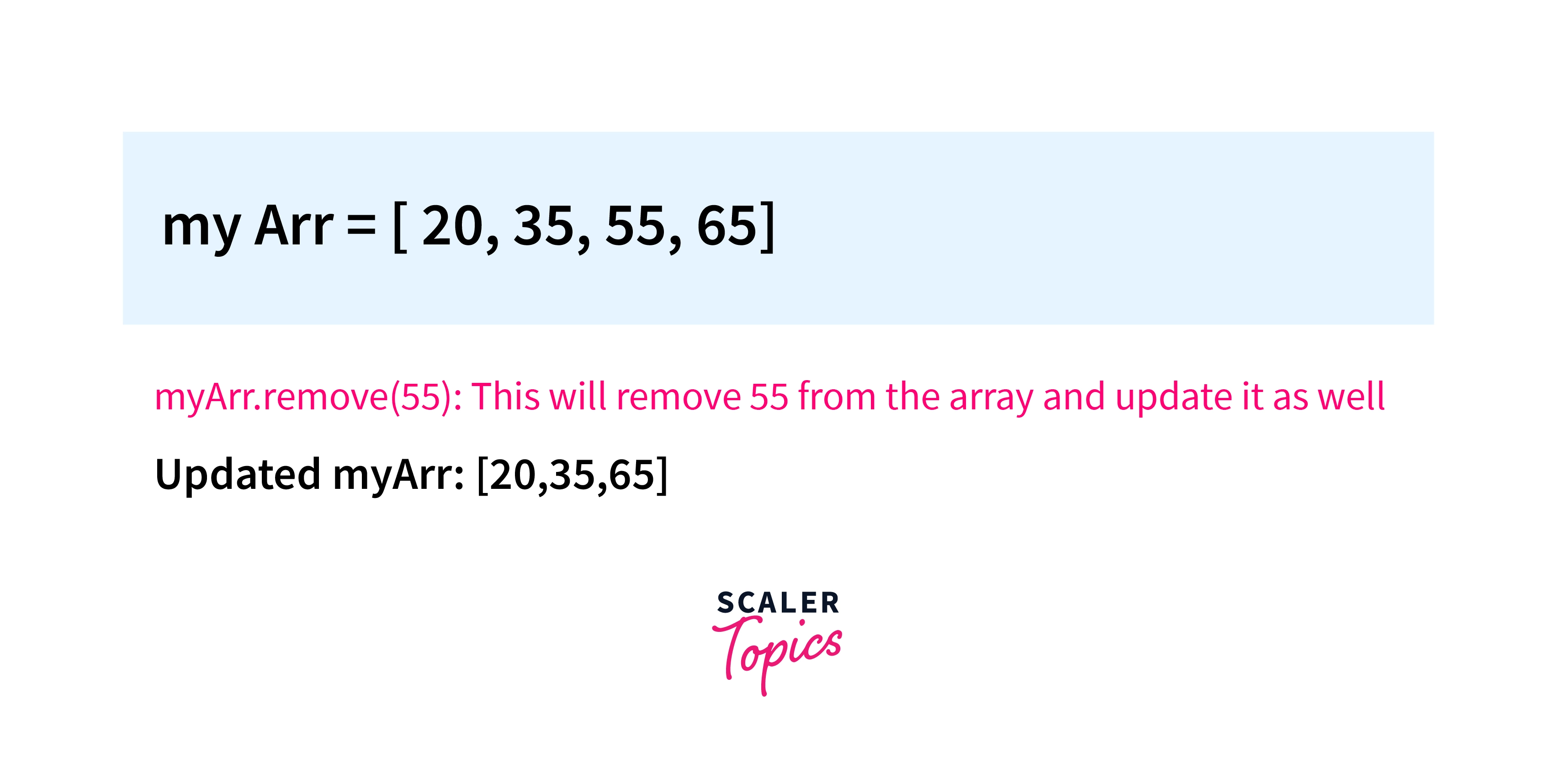Syntax

Example

This code removes the element 55 from the existing array and re-arranges the array at the same time.

Output

The above code removes 55 from the array and prints the updated array as the output.

Explanation

First of all, we have imported the array class into the code and then create an array. After that, we have used .remove() and .pop() function for removing a specific element from the array. Finally, we printed the array to get the desired output.

## Searching Elements from Array in Python

From the sentence "searching an element from the Array" this means we have to find the index of the particular element of the array.

• This can be achieved with the help of .index() which returns the index of the element which is present in the array.
• If we have duplicate elements whose index is to be calculated then the .index() will return the index of the first occurrence of that element.
• if we passed an element that is not present in the array as a parameter to the index() then it will raise the ValueError i.e. the particular element is not present in the array.Syntax

Example

This code finds the position/index of the searched element in the array.

Output

The above code prints the index of the element which is searched inside it.

Explanation

First of all, we have imported array class into our code. Then, we create the array with the array function of the class which we have imported earlier. At last, we have used index() on the array which returns the index of the element which we have entered as a parameter to the function and then prints it on the output screen.

## Updating Elements in Array in Python

For updating a value/element in an array we just need to re-assign a new value at the desired index where we want to update the old value with the new one.

Note: If we are trying to update the element whose index is greater than the last index of the array then it will raise the error i.e. index out of range or in general terms we can say that index is out of bounds.

Example

This code will update the value which is present at index 2 with 100.

Output

The above code updates the value which is present at the 2nd index of the array with 100 and prints it as an output.

Explanation

First of all, we have imported array class into our code. Then, we create the array with the array function of the class which we have imported earlier. Then we use the rectangular brackets to update the value at a particular index with a new value. In the end, we print the updated array on our output screen.

## Reverse an Array

Python includes a built-in function that is used to create a reverse iterator: the reverse() function. This iterator works because arrays are indexed, so each value in an array can be accessed individually. The reverse iterator is then used to iterate through the elements in an array in reverse order.

So, For reversing an array we just need to use the .reverse() function which flips the array.

Example

In this code, we used to reverse() to reverse the array.

Output

The above code reverses the array with the help of .reverse() and prints it as an output.

Explanation

First of all, we have imported array class into our code. Then, we create the array with the array function of the class which we have imported earlier. Finally, we have used the reverse function on the array which reverses the array and then prints the reversed new array on the output on the output screen.

Example

Let's assume you have a marks array in which you have stored marks of 5 subjects. You have to update the array where marks are 20 and then update it with 90 marks.

Let's write the code to do so.

Code

This code updates the 20 marks with the 90 marks.

Output

## Slicing of an array

Slicing in Python allows you to extract a portion of an array, list, or string by specifying a range of indices. It provides a concise and efficient way to access specific elements or subarrays within a larger sequence. The basic syntax for slicing is start:stop, where:

• start: The index where the slice starts (inclusive). If not specified, it defaults to the beginning of the sequence.
• stop: The index where the slice ends (exclusive). If not specified, it defaults to the end of the sequence.
• step: The interval between elements to be included in the slice. If not specified, it defaults to 1.

Example:

Output:

Let's see another example in which we will try to slice a list using step

Example:

Output:

## Counting Elements in a Array

To count the number of elements in an array in Python, you can use the built-in len() function. The len() function returns the number of items in a sequence, such as an array or a list. Here's how you can use it:

Output:

## Array methods in Python

MethodDescription
append()Adds an element to the end of the array.
extend()Appends elements from an iterable to the end of the array.
insert()Inserts an element at the specified position in the array.
remove()Removes the first occurrence of a specified element from the array.
pop()Removes and returns the element at the specified position.
index()Returns the index of the first occurrence of a specified element in the array.
count()Returns the number of occurrences of a specified element in the array.
sort()Sorts the array in ascending order.
reverse()Reverses the order of elements in the array.
copy()Returns a shallow copy of the array.

## Conclusion

• First of all, we understand what are arrays in python and understand the different properties of arrays in python.
• Then we go through the steps that how we can create an array, how can we access elements of the array, and much more.
• At the end, we made a case in which we have stored marks of a student in an array and we are updating that array with the array methods.